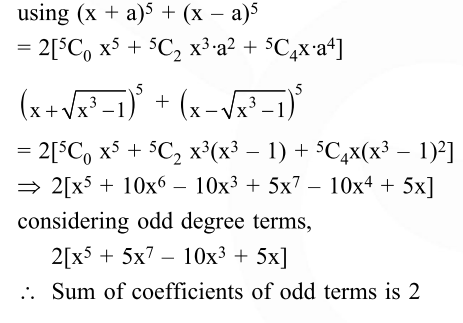# The sum of the co-efficients of all odd degree terms in the expansion of`
Question:

The sum of the co-efficients of all odd degree terms in the expansion of $\left(x+\sqrt{x^{3}-1}\right)^{5}+$ $\left(x-\sqrt{x^{3}-1}\right)^{5},(x>1)$ is-

1. 0

2. 1

3. 2

4. 5

Correct Option: , 3

Solution: